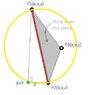# Find point coordinate on 3D circle knowing three points

alaam2005
I would like to find a 3D coordinate of a point (X) on a circle, knowing two points on the circle (P1,P2) which represent the circle diameter and another point (P3) NOT on the circle but on its plane. Also known the length of the line from P2 to X, for example d. Another thing that may help, the line from the point P1 to X is perpindicular to the line X to P2.
Declared figure is attached.

Any help is appreciated.

#### Attachments

•Circle_3P.jpg
12.8 KB · Views: 415

Hey alaam2005 and welcome to the forums.

The easiest way to do this is to construct the plane and get an equation for the plane and then find the intersection of a plane with a sphere.

The plane can be constructed by getting the normal vector to be (P2-P1) X (P3-P1) [Cross Product] and then using the fact that n . (r - r0) = 0 where r0 is a point on the plane to get your plane equation ax + by + cz + d = 0.

You then have an equation for your sphere which is (x-a)^2 + (y-b)^2 + (z-c)^2 = 0 (different a, b, and c for sphere and plane equations). Equate the two and you will get an equation in two variables which will give you an equation for the circle that cuts the plane.

Now you use your distances from P1 and P2 to the point and collect everything together to get an answer.

alaam2005
Thanks for fast response chiro
After I posted this I searched this forum for related issues and got some answers which is close to your answer. However, when I apply it I don't get feasible results.Assuming P4 as the point to find, similar to plane eqn you mention it, I used, a norm vector as
N=cross(P2-P1,P2-P3), where cross is cross product
then normalize it,
N/norm(N).
dot(P4-P2,N)=0 ...(1) where dot means dot product.
and got the plane equation. Having length d,
norm(P4-P2)=d ...(2)
which will contain sequared variables. Also, I have
dot(P4-P2,P4-P1)=0...(3)
cause the line P4P1 should be normal to P4P2, and ths equation will contains sequared variables. Subtracting eqn (2) from (3) I will get a second linear equation.
Any point on the sphere will be true, so my point P4 is applied to the sphere equation which need (Pc) center of sphere and its radius (r).
Pc=(P1+P2)/2 and r=norm(P1-Pc), correct?
so,
norm(Pc-P4)=r ...(4)
which will contains squared variables. Subtracting (4) from (2) will get the third linear equation. Solving the 3 linear equations to get P4(x,y,z) gave not feasible answers.
May I get help on this please, did I have to normalize other vectors?, my background in vectors is not that much and all I knew learned from your forum. Any wrong in this procedure. Thanks in advance.

Last edited:
Your plane should be based on calculating (P2-P1)X(P3-P1) (i.e you have the same point being deducted in both terms). Also for the equation I'm not sure if the . dot means just a dot product or something else.

For dot(P4-P2,P4-P1) your heads of the vectors are P2 and P1 so you reverse the order to be dot(P2-P4,P1-P4)

So let's look at how many equations you have:

<N,(P4 - P2)> = 0 which gives the equation for a circle.
<P4-P2,P4-P2> = d^2 which reduces it down to 2 choices
<P2-P4,P1-P4> = 0 which reduces it down to 1.

Since you have the constraint (x-a)^2 + (y-b)^2 + (z-c)^2 = r^2. (where (x,y,z) = P4).

You don't need the rest of the other stuff: maybe you should show your calculations in detail just for clarification.

alaam2005
Your plane should be based on calculating (P2-P1)X(P3-P1) (i.e you have the same point being deducted in both terms). Also for the equation I'm not sure if the . dot means just a dot product or something else.

For dot(P4-P2,P4-P1) your heads of the vectors are P2 and P1 so you reverse the order to be dot(P2-P4,P1-P4)

So let's look at how many equations you have:

<N,(P4 - P2)> = 0 which gives the equation for a circle.
<P4-P2,P4-P2> = d^2 which reduces it down to 2 choices
<P2-P4,P1-P4> = 0 which reduces it down to 1.

Since you have the constraint (x-a)^2 + (y-b)^2 + (z-c)^2 = r^2. (where (x,y,z) = P4).

You don't need the rest of the other stuff: maybe you should show your calculations in detail just for clarification.

Yes chiro, the dot means dot product and I added meaning of it to my previous reply, thanks for reminding about it.
I have to disagree with you concerning the order in the dot product cause at end it will equals zero. So It's order will not affect the result.
Concerning the equations, you mentioned 4 equations which are the 4 equations I also mentioned above. The equations which includes X^2,Y^2,and Z^2 it will be harder to solve so I added the 4th equation to remove them using subtraction.
My concern is, why the N vector is only normalized while other vectors not? I'm afraid the error is concerning normalizing another vector !

Now, I'm trying another way, by using the method which relates to 3 points on a circle and finding the center of the circle. My 3 points will be P1,P2,P4 so when I find the center of the circle using these points I will have 3 equations and I will equalize it to the center of the circle which I already have it, Pc. But its too long that I may have to solve it numerically (like Newton Raphson) to solve them!